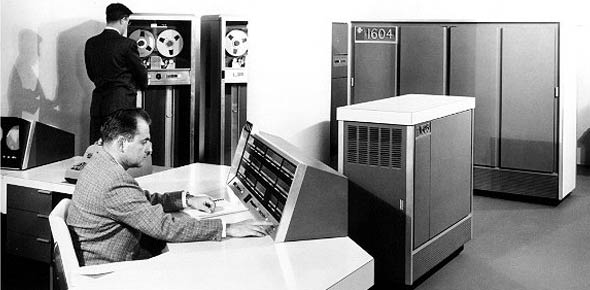# 3C051 CDC Vol. 1

73 Questions | Attempts: 1115
ShareSettingsThis is all URE questions from Volume 1 of the 3C051 CDC (As of 2009)

• 1.
(001) What is the first job the basic input/output system (BIOS) does at start up?
• A.

Run memory byte count.

• B.

Test for parity errors.

• C.

Load instructions to random access memory (RAM).

• D.

Run the power-on self-test.

• 2.
(001) Which original component was very susceptible to damage from electrostatic discharge?
• A.

• B.

Basic input/output system (BIOS).

• C.

Complementary metal-oxide semiconductor (CMOS).

• D.

Electrically erasable programmable read-only memory (EEPROM).

• 3.
(001) Which component uses a lithium battery to maintain computer settings when power is removed?
• A.

• B.

Basic input/output system (BIOS).

• C.

Complementary metal-oxide semiconductor (CMOS).

• D.

Electrically erasable programmable read-only memory (EEPROM).

• 4.
(001) What is considered as nonvolatile permanent memory?
• A.

• B.

Basic input/output system (BIOS).

• C.

Complementary metal-oxide semiconductor (CMOS).

• D.

Electrically erasable programmable read-only memory (EEPROM).

• 5.
(001) What is a form of firmware that contains the computer's startup instructions?
• A.

Basic input/output system (BIOS).

• B.

Complementary metal-oxide semiconductor (CMOS).

• C.

Cache.

• D.

Electrically erasable programmable read-only memory (EEPROM).

• 6.
(001) What static RAM (SRAM) uses special applications in a CPU?
• A.

Basic input/output system (BIOS).

• B.

Complementary metal-oxide semiconductor (CMOS).

• C.

Cache.

• D.

Electrically erasable programmable read-only memory (EEPROM).

• 7.
(001) On most modern computers, which has two or three levels of memory?
• A.

Complementary metal-oxide semiconductor (CMOS).

• B.

Cache.

• C.

Double Data Random Access Memory (DDRAM).

• D.

Electrically erasable programmable read-only memory (EEPROM).

• 8.
(001) Actively sampling the status of an external device by a client program is know as
• A.

Polling

• B.

Signaling

• C.

Processing

• D.

• 9.
(002) What is defined as an asynchronous signal from a computer hardware device indicating the need for attention from a central processing unit (CPU) or a synchronous event?
• A.

Cache

• B.

Buffer

• C.

Interface

• D.

Interrupt

• 10.
(002) What interrupt request (IRQ) line number is normally used for the floppy disk controller?
• A.

2

• B.

4

• C.

6

• D.

8

• 11.
(002) Which term describes that data is whole or complete?
• A.

Driver

• B.

Parity bit

• C.

Data integrity

• D.

Error correction

• 12.
(002) What is considered as a technique or method of checking data?
• A.

Asynchronous

• B.

Parity bit

• C.

Interrupt

• D.

Fault

• 13.
(002) Which is a very simple example of an error detecting code?
• A.

Interrupt

• B.

Parity bit

• C.

Asyncronous

• D.

Data integrity

• 14.
(002) What component initializes communication with all hardware devices and sends a message if a keyboard or mouse is not found?
• A.

• B.

Basic input output system (BIOS)

• C.

Complementary metal-oxide semiconductor (CMOS)

• D.

Electrically erasable programmable read-only memory (EEPROM)

• 15.
(002) Which is a small, low-level program used by the computer operating system to interact with hardware devices?
• A.

Basic input output system (BIOS)

• B.

Driver

• C.

Parity

• D.

Interrupt

• 16.
(003) What is the sudden and momentary electric current that flows between two objects at different electrical potentials?
• A.

Voltage

• B.

Amperage

• C.

Conductivity

• D.

Electrostatic discharge

• 17.
(003) Static electricity remains intact until it is
• A.

Absorbed

• B.

Dissolved

• C.

Discharged

• D.

Neutralized

• 18.
(003) Under what class of electrostatic discharge (ESD) would an item fall if it was sensitive to 900 volts?
• A.

I

• B.

II

• C.

III

• D.

IV

• 19.
(003) Electrostatic discharge (ESD) items sensitive to more than 4,000 but less than 15,000 volts fall into category
• A.

I

• B.

II

• C.

III

• D.

IV

• 20.
(003) What measure would you take to prevent the build up of static electricity?
• A.

Ground the electrostatic discharge sensitive (ESDS) device

• B.

Check the relative humidity

• C.

Disconnect the circuit from the device

• D.

Turn the power switch to the off position

• 21.
(003) What is the first step you must take when either installing or removing an electrostatic discharge sensitive device (ESDS)?
• A.

Ground the ESDS device

• B.

Check the relative humidity level

• C.

Disconnect the circuit from the device

• D.

Turn the power switch to the off position

• 22.
(004) The process of collecting and analyzing data to determine the cause of an operational failure and how to prevent it from recurring is called
• A.

Fault isolation

• B.

Event evaluation

• C.

Process investigation

• D.

Analytical consideration

• 23.
(004) In which troubleshooting step would you determine which conditions are present?
• A.

Define the problem

• B.

Isolate the problem

• C.

Resolve the problem

• D.

Confirm the resolution

• 24.
(004) In which troubleshooting step would you confirm a dilemma exists?
• A.

Define the problem

• B.

Isolate the problem

• C.

Resolve the problem

• D.

Confirm the resolution

• 25.
(004) In which troubleshooting step would you identify likely causes and eliminate unlikely causes?
• A.

Define the problem

• B.

Isolate the problem

• C.

Resolve the problem

• D.

Confirm the resolution

## Related TopicsBack to top
×

Wait!
Here's an interesting quiz for you.